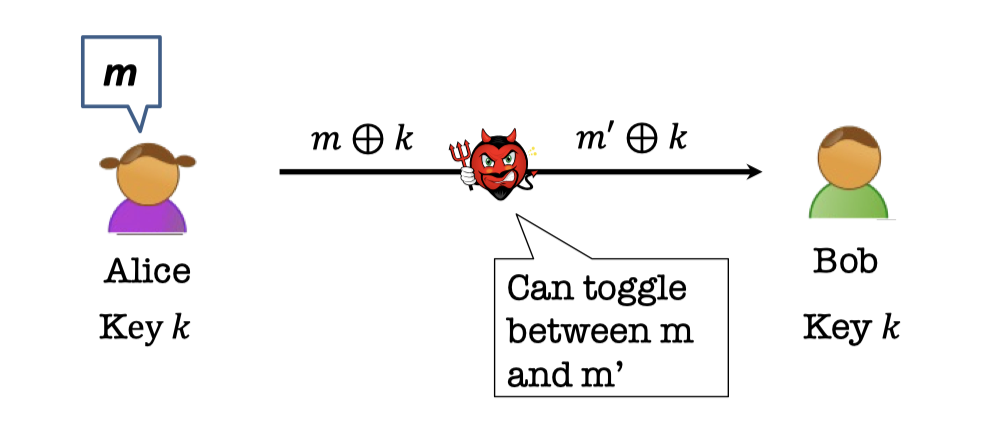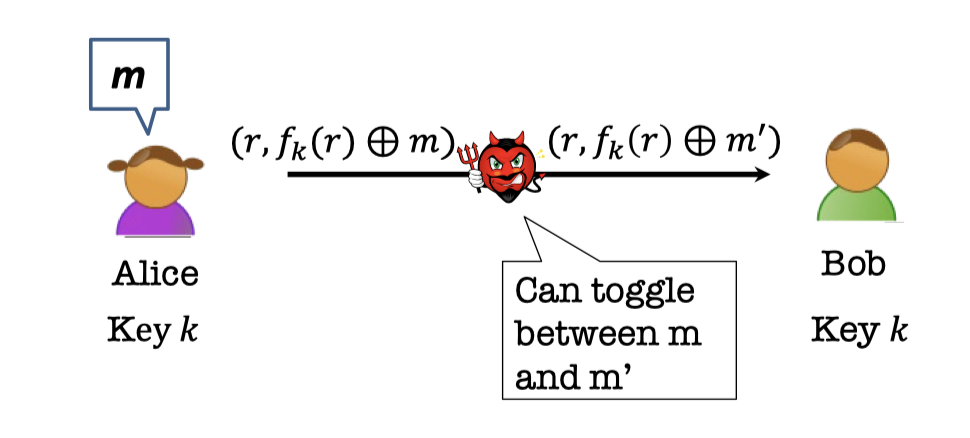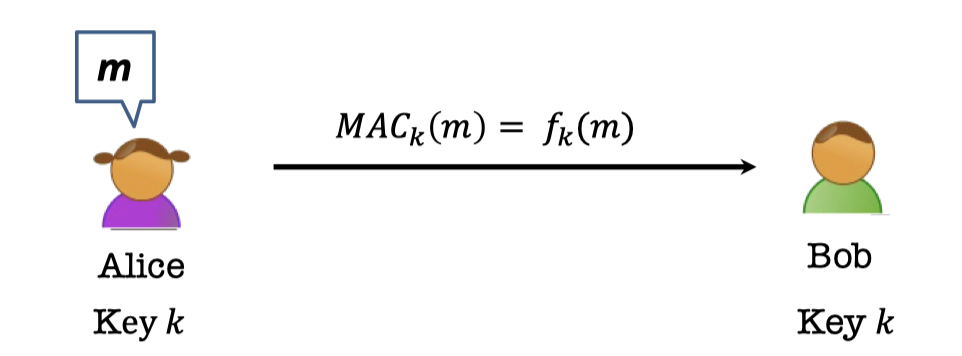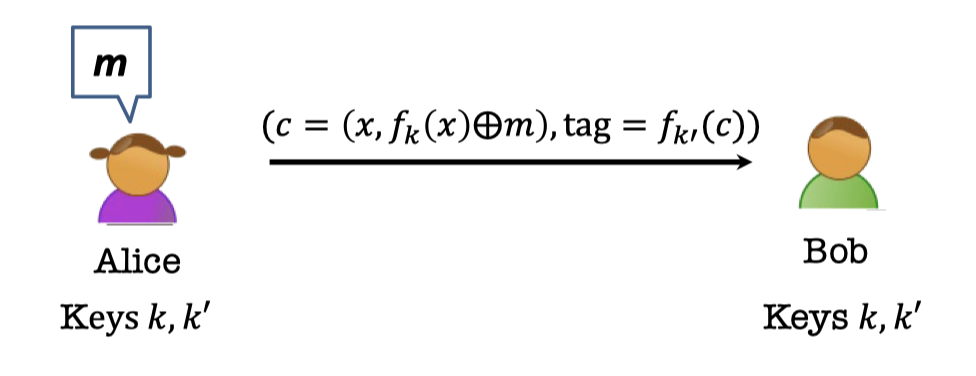# 「Cryptography-MIT6875」: Lecture 5

In this series, I will learn MIT 6.875, Foundations of Cryptography, lectured by Vinod Vaikuntanathan.
Any corrections and advice are welcome. ^ - ^

Topics covered:

• Applications of PRFs

Note: The second half of Lecture 5, Number Theory, is contained in Lecture 6.

# Recap

In last blog, we introduced the security definition of the stateless secret-key encryption.

Then we elaborated on the theorem of PRF, if there are PRGs, then there are PRFs, and gave the Goldreich-Goldwasser-Micali (GGM) construction.

# Applications of PRFs

In this blog, we will show some applications of PRF.

• Identification Protocols
• Authentication
• Application to Learning Theory.

## Identification Protocols

PRF is widely used in identification protocols.

Think about the situation that you take your ID card and put it on a RFID to pay the money.

The ID card has a secret embedded in it that is specific to your cost right. So it’s sort of a key that the card contains.

The device has access to a database which stores all the students keys.

The student need the ID card to authenticate to the device.

However, there is an eavesdropper in the channel, who is listening to the communications and wants to impersonate Tim.PRFs can prevent the adversary from impersonating.

### Unpredictability of PRF

Before that, let’s introduce a simple lemma about unpredictability of PRF.

Let $f_s:\{0,1\}^l\rightarrow \{0,1\}^m$ be a pseudorandom function.

Consider an adversary who requests and obtains $f_s(x_1),\dots,f_s(x_q)$ for a polynomial $q=q(n)$.

Can she predict $f_s(x^*)$ for some $x^*$ of her choosing where $x^*\notin \{x_1,\dots, x_q\}$?

Lemma:

If she succeeds with probability $\frac{1}{2^m}+1/p(n)$, then she broke PRF security.
This $(\frac{1}{2^m})$ is negligible in $n$ is $m$ is large enough.

It’s easy to comprehend that the adversary cannot even distinguish it and certainly she cannot predict it.

So indistinguishability implies unpredictability and it turns out the lemma is right.

In [the lecture 3], we proved that the Unpredictability = Indistinguishability for bits (or, for PRG).

However, for PRF, Indistinguishability → Unpredictability, but not vice versa.

### Challenge-Response Protocol

The secret in the ID card is the PRF key $s$.

The device has access to the database which stores all the students’ mapping relation, (ID number $ID$, PRF Key $s$).

Whenever Tim wants to authenticate to the device, the protocol is as follows:

1. The device sends a random $r$ to the ID card.
2. The ID card use the embedded key $s$ to evaluate $f_s(r)$, and sends the pair $(ID, f_s(r))$ to the device.According to the lemma of unpredictability, it’s easy to prove the security of the protocol.

The adversary collects $(r_i, f_s(r_i))$ for poly. many $r_i$(potentially of her choosing).

She eventually has to produce $f_s(r^*)$ for a fresh random $r^*$ when she is trying to impersonate.

This is hard as long as the input and output lengths of the PRF are long enough.

## Authentication

Another scenario is to use PRF as the Message Authentication Code(MAC).

Consider the initial secure communication.

Alice and Bob have an agreed key $k$, and they use the one-time pad to encrypt the message.

The adversary can learning nothing from the ciphertext according to Perfect Secrecy.

But one-time pad (and encryption schemes in general) are malleable. The adversary can toggle between $m$ and $m’$ easily. She can change the valid ciphertext $m\oplus k$ to a totally different ciphertext $m’\oplus k$.Likewise, the adversary can change the valid ciphertext $(r, f_k(r)\oplus m)$ to a totally different ciphertext $(r,f_k(r)\oplus m’)$ in the stateless secret-key encryption.### MAC

It is of importance to use Message Authentication Codes.

Alice and Bob have an agreed key $k$ to specify a pseudorandom function $f_k$.

MAC is evaluated by the pseudorandom function $f_k$, the message $m$ taken as input.

People can see a bunch of messages and tags (MAC) and cannot come up with the new message and the corresponding tag.MACs give us integrity, but not privacy.

There is a solution to guarantee the privacy, Encrypt, then MAC.

Note that there are two difference PRF $f_k$ and $f_{k’}$ since the input length is different.## Learning Theory

There is a negative results in learning theory.

Theorem [Kearns and Valiant 1994]:

Assuming PRFs exist, there are hypothesis classes that cannot be learned by polynomial-time algorithms.

It’s showed that the function cannot be learned if PRFs exist.

But it gives a counterpoint to machine learning that says everything can be learned. You just throw the samples to a deep neural network.

「Cryptography-MIT6875」: Lecture 5

https://f7ed.com/2022/07/12/mit6875-lec5/

f1ed

2022-07-12

2022-08-12